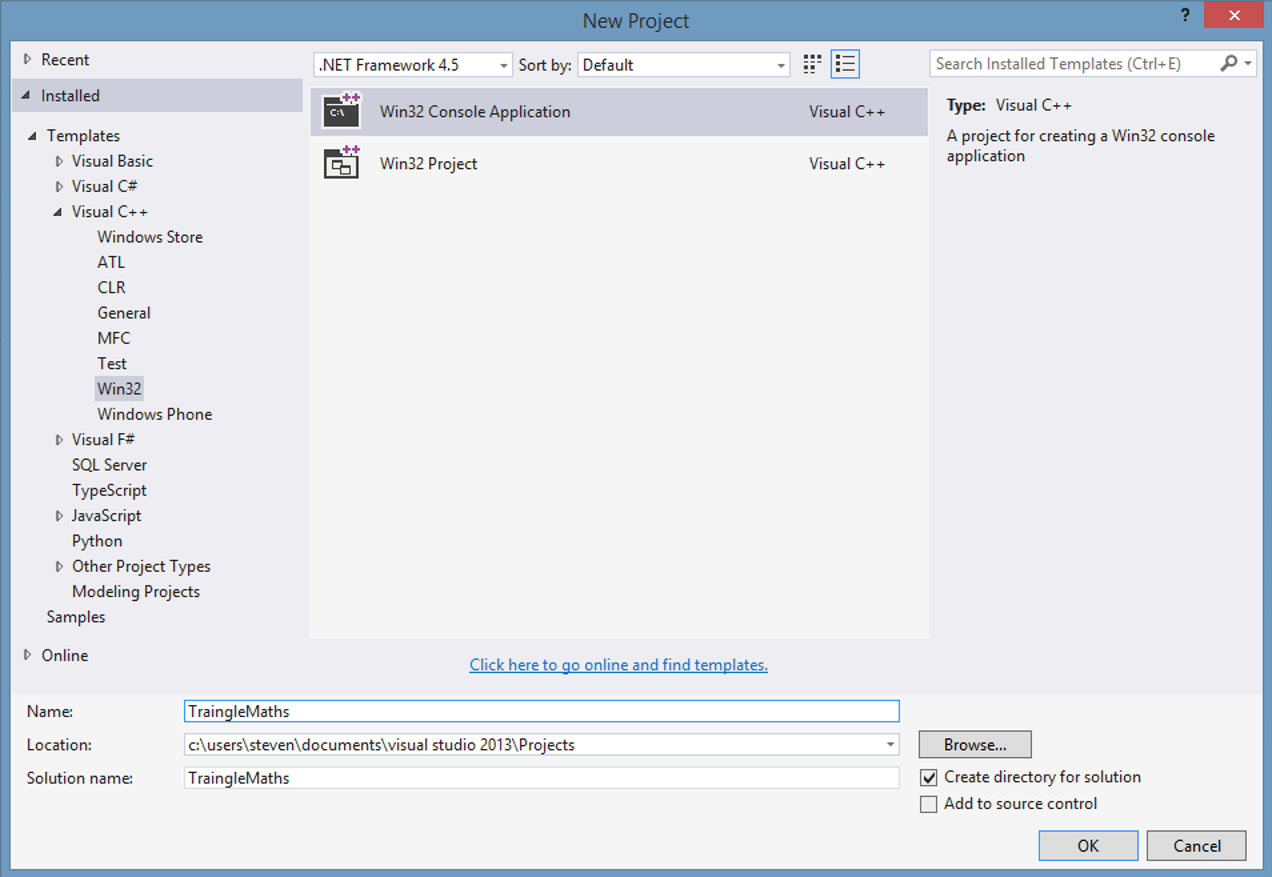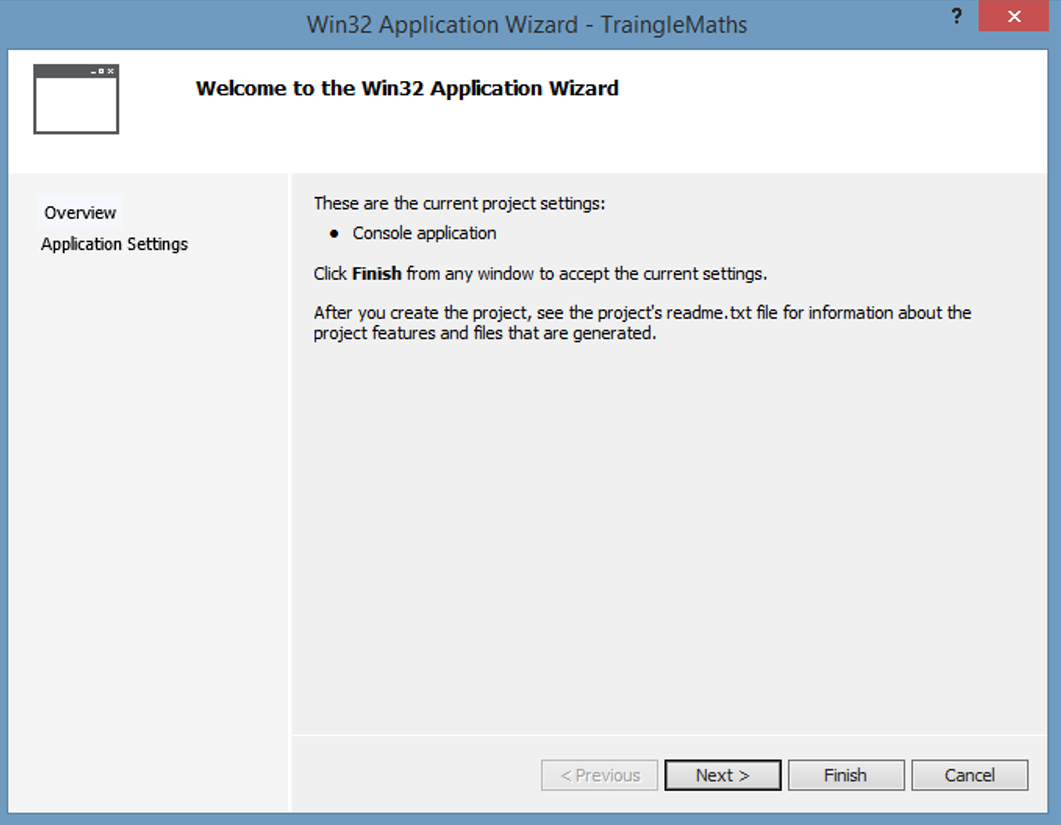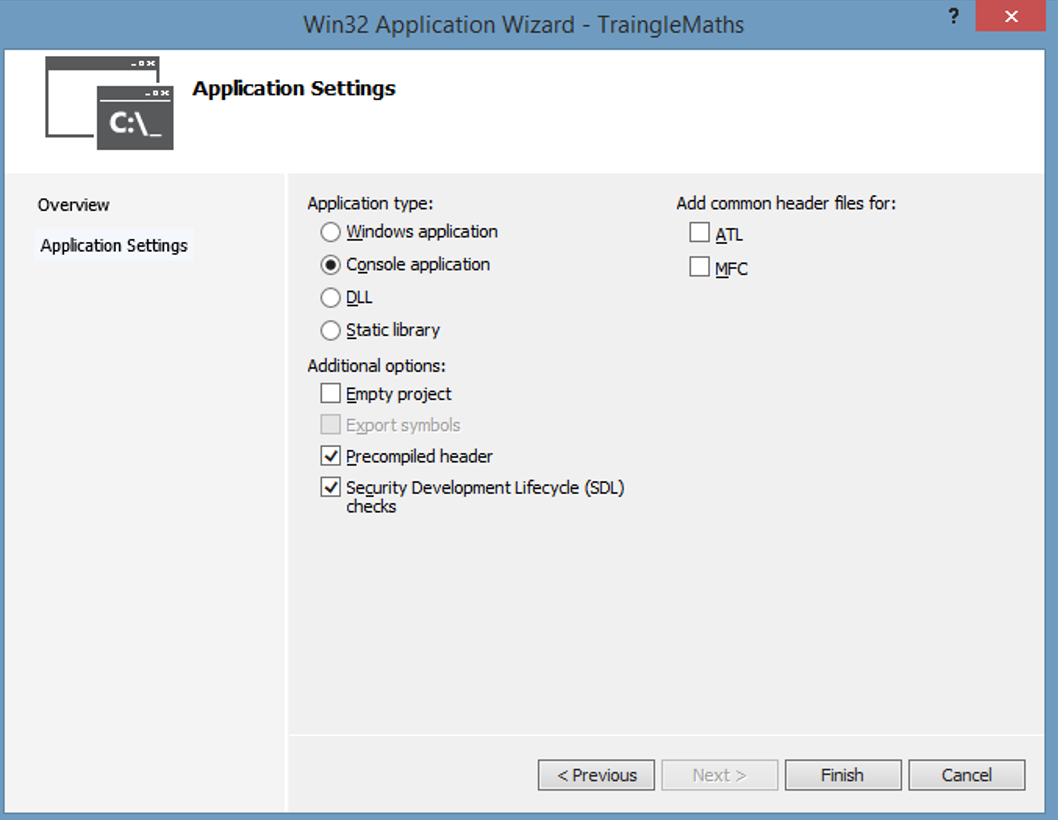# 1. C++ language fundamentals

## Tutorial 1: Introduction to C++

1. Setting the Scene
• C++ is an object-oriented evolution of C
• C dates back to 1972
• C++ was introduced in 1985, by Bjarne Stroustrup
• C++ is now an ANSI standard
• Characteristics of C++
• Object-oriented, i.e. supports es, ,
• Strongly typed at compile-time
• Supports generic programming via templates, e.g. list<int>
• Uses of C++ are extremely widespread
• E.g. middle-tier business rules in a distributed application
• E.g. low-level process control
2. Differences between C++ and Java
• C++ is compiled directly to machine code
• No concept of “byte codes” (like you have in Java)
• So you must re-compile for each target platform
• C++ applications run directly on the O/S
• No virtual machine between C++ and the O/S
• No – you must de-allocate objects yourself
• The C++ Standard Template Library (STL) is extremely limited compared to the Java SE library
• STL has string, IO, and collection classes
• But STL doesn’t have GUI / database / network / multithreading!
3. The Standard C++ Library
• The standard C++ lib incorporates the standard C lib
• You include files such as <cstdio>, <cmath>, etc.
• Declarations are nested in the std namespace, so you can use a using namespace statement to bring into scope
```#include <cstdio> // #include is a pre-processor directive to include a header file. #include <cmath> using namespace std; // Allows unqualified access to standard C++ functions and classes. ```
• The standard C++ lib also defines standard C++ classes
• You include files such as <iostream>, <vector>, etc.
• Declarations are nested in the std namespace, so you can use a using namespace statement to bring into scope
```#include <iostream> #include <vector> using namespace std; ```
4. Hello World!
• Here’s a traditional “Hello World” program in C++
• main() is a global , the entry-point in a C++ application
• cout outputs a message to the console (there’s also cin, cerr)
• endl means “new line”
```// Include the standard header file that declares cout and endl. #include <iostream> // Allow easy access to cout and endl, in the std namespace. using namespace std; int main() {   cout << "Hello world!" << endl;   return 0; } ```
• You can also declare main() as follows
```int main(int argc, char *argv[]) ... ```
• C++ is a compiled language
• Comprises source-code files (e.g. Account.cpp - you can name files anything you like!)
• Source-code files are compiled to object files (e.g. Account.obj)
• Object files are linked together, along with library files perhaps, to create executable files (e.g. MyApp.exe)
• For example, to compile/link a C++ program in Linux:
• You can use the g++ command-line compiler/linker
• Use the -o option to specify the name of the executable output file
• Here's an example...
• Compiles HelloWorld.cpp to an object file
• Links the object file with the standard C++ library, to create an executable output file named Hello
```g++ HelloWorld.cpp -oHello ```
```man g++ ```
6. Getting Input
• string is a standard C++ class, defined in the <string> header
• cin inputs a value (e.g. a string or an int) from the console
• Here's an example of how to get input from the console
```#include <iostream> #include <string> using namespace std; int main() {  string name;  int age;  cout << "Hi, what's your name? ";  cin >> name;  cout << "How old are you? ";  cin >> age;  cout << name << ", on your next birthday you'll be " << age + 1 << endl;  return 0; } ```
7. Making Decisions
• Here's an example of making simple decisions
```#include <iostream> #include <cmath> using namespace std; int main() {  int a, b, c;  cout << "Enter the coefficient of x-squared, x, and units: ";  cin >> a >> b >> c;  double disc = (b * b) - (4 * a * c);  if (disc < 0)  {   cerr << "Roots are imaginary " << endl;  }  else  {   double root1 = (-a + sqrt(disc)) / 2 * a;   double root2 = (-a - sqrt(disc)) / 2 * a;   cout << "Roots are " << root1 << " and " << root2 << endl;  }  return 0; } ```

#### Lab

1. Creating a C++ application
• Start Microsoft Visual 2013, and invoke the File | New | Project menu command. In the New Project dialog box, create a new C++ application project named TriangleMaths in the student folder, as follows:
•• This will create a C++ Win32 Console Application, i.e. a regular C++ application that doesn't use any of Microsoft's C++ libraries or .NET features. Click OK.
• Another dialog box appears; this is the first screen in the "Win32 Application Wizard":
•• Click Next to move on to the next step in the wizard.
• The next screen in the wizard allows you to configure the project. You can just click Finish to accept the default options (if you're interested, see the notes on the following page for a quick explanation of what all these options mean):
•• Here's a brief explanation of the Application Settings options from the dialog box on the previous page (the items in bold are a reminder of the options you selected)...
• Application type:
• Windows application - creates a GUI application using the Windows SDK
• Console application - creates a console application that runs in a command window
• DLL - creates a .dll dynamic link library that can be shared by many applications
• Static library - creates a .lib library that can be embedded in individual applications
• Empty project - creates an empty project with no source code
• Export symbols - this option is only available to DLL projects. A .dll file has a layout very similar to an .exe file, with one important difference - a DLL contains an exports table. The exports table contains the name of every that the DLL exports to other executables. These s are the entry points into the DLL; only the s in the exports table can be accessed by other executables (any other s in the DLL are private to the DLL)
• Precompiled header - Some header files can be extremely large. For example, many commercial C++ libraries such as Boost and POCO implement most of their functionality in header files (this is because of the way templates work in C++ - see later in the course for details). These header files would have to be recompiled every time they are included in source files! To reduce times, some compilers allow header files to be compiled into a form that is faster for the compiler to process. This intermediate form is known as a precompiled header, and is commonly held in a file named with the extension .pch (as in Visual C++) or .gch (as in the GNU compiler on Linux)
• ATL - Active Template Library is a set of template-based C++ classes from Microsoft, intended to simplify COM programming. COM has been largely superseded by the .NET Framework (although many of the internal components in Windows are still implemented using COM!)
• MFC - Microsoft Foundation Classes is a large framework from Microsoft, intended to simplify GUI programming. MFC has been entirely superseded by the .NET Framework

## Tutorial 2: Basic syntax rules

1. Statements and Expressions
• C++ code comprises statements
• C++ statements end with a semi-colon
• You can group related statements into a block, by using {}
• C++ code is free-format
• But you should use indentation to indicate logical structure
• An is part of a statement. For example:
• a + b
• a == b
• Single-line comment
• Use //
• Remainder of line is a comment
• Block comment
• Use /* ... ... */
• Useful for larger comments, e.g. at the start of an algorithm
• Note:
• So you can't use /** ... ... */
3. Legal Identifiers
4. Classes
• You can define classes (nouns) in any source file
• You don't have to define classes in a file with the same name
• ...although many developers do like to do this
• Standard C++ classes are all lowercase
• E.g. string
• E.g. list<T>
• Some developers like to use capitalization (like in Java)
• E.g. BankAccount
• E.g. Customer
5. Functions and Methods
• C++ is based on C (this is an important pragmatic point)
• So C++ supports global s
• i.e. you don't have to define everything in a class!
• C++ offers many global functions from its C heritage, and are all lowercase
• E.g. sqrt()
• E.g. printf()
• Some developers like to use capitalization
• E.g. CalcInterest()
• E.g. TransferFunds()

#### Lab

1. Understanding Microsoft Visual C++ projects
• In Solution Explorer, Visual Studio has created various header files and source files. First expand the Header Files folder and take a look at these header files:
• stdafx.h - This header file will be precompiled by the Microsoft compiler. Therefore, if you have any large header files that you want to include in many source files, you should put the #include statements in here. This will ensure that the headers are compiled only once, rather than being recompiled every time you build the project
• targetver.h- This header file specifies the target version of Windows for your application. You will probably never need to change this file
• Now expand the Source Files folder and take a look at these files:
• stdafx.cpp - This source file just includes the stdafx.h header. This source file is compiled to create the precompiled header file for the project. You'll never need to change this file
• TriangleMaths.cpp - This is the main source file for the project, and it's where you'll add all your source code in this lab. Note that the entry point function is _tmain() and it mentions _TCHAR in the argument list... these are Microsoft-specific macros that allow you to support either 8-bit characters or 16-bit wide characters, based on project settings. You can ignore the details for now, we'll discuss it later!

## Tutorial 3: Declaring and using variables

1. Variables
• All applications use s
• A variable has a name, a type, and a value
• Note, all variables in C++ are "garbage" until you assign a value!!!
• General syntax:
```type variableName = optionalInitialValue; ```
• Example:
```int yearsToRetirement = 20; ```
• A variable also has a scope:
• Block scope
• Method scope
• Object scope
• Class scope
2. Constants
• A constant is a fixed "variable"
• Use the const (similar to final variables in Java)
• Make sure the constant has a known initial value
• The compiler will ensure you don't modify the constant thereafter
• General syntax:
```const type CONSTANT_NAME = initialValue; ```
• Example:
```const long SPEED_OF_LIGHT = 299792458; const int SECONDS_IN_MIN = 60; const int SECONDS_IN_HOUR = SECONDS_IN_MINUTE * 60; const string BEST_TEAM_IN_WALES = "Swansea City"; ```
3. #define Directives
• C++ code can use #define directives (originated in C)
• Anything that starts with # is a pre-processor directive
• Expanded (prior to ) by the pre-processor
• Examples in the <climits> standard C++ header file:
```#define INT_MIN -32767 #define INT_MAX 32767 #define UINT_MAX 65535 ... ```
• #define directives can take s
• These are known as macros... good or bad?
```#define MAX(a, b) (((a) > (b)) ? (a) : (b)) ```
4. C++ Built-in Types
• Here is the full set of built-in types in C++ - Note: the size/range details vary, based on compiler and platform:
5. Using Integers
• Integers store whole numbers
• C++ supports signed and unsigned ints (default is signed)
• Absolute size of these types is platform-dependent!
• You can use decimal, hex, or octal notation
• Examples:
```int x, y, z; short age; long population; unsigned short goalsScored; int yearOfBirth = 1997; short goalDifference = -5; long favouriteColor = 0xFF000000; // Hexadecimal int bitMask = 0701; // Octal ```
6. Using Floating Point Variables
• Floating point variables store fractional and large values
• float holds a single-precision floating point number
• double holds a double-precision floating point number
• Should you use float or double?
• Use double in most cases (all the C++ s do)
• ...unless memory is critical
• Examples:
```double pi = 3.14159; double c = 2.99E8; // 2.99 x 108 double e = -1.602E-19; // -1.602 x 10-19 float height = 1.58F; // The F (or f) suffix means "float", i.e. not "double". float weight = 58.5F; // Ditto ```
7. Using Characters
• Characters are single-byte values (not Unicode!) - Enclose character value in single quotes
• Examples:
```char myInitial = 'A'; char nl = '\n'; // Newline char cr = '\r'; // Carriage return char tab = '\t'; // Horizontal tab char nul = '\0'; // Null character (ASCII value 0) char bsl = '\\'; // Backslash char sqt = '\''; // Single quote char dqt = '\"'; // Double quote char aDec = 97; // Assign decimal value to char char aOct = '\141'; // Assign octal value to char char aHex = '\x97'; // Assign hexadecimal value to char ```
• If you want to store Unicode characters, use wchar_t
```wchar_t unicodeChar = L'A'; ```
8. Using Booleans
• bool variables are true/false values
```bool isWelsh = true; bool canSing = false; ```
• C++ supports automatic conversions to bool
• Any non-zero value implicitly means truth
```int errorCount; ... bool isBad = errorCount; // If errorCount is non-zero, isBad is true. cout << isBad; // Outputs true or false. ```
• C++ allows non-booleans in test conditions
• Quite different than Java!
```int errorCount; ... if (errorCount) { ... } // If errorCount is non-zero, it means "true". ```

#### Lab

1. Performing input and output
• Modify TriangleMaths.cpp as follows:
• Add a #include statement, to include the <iostream> standard header file.
```#include <iostream> ```
• Add a using namespace std statement, to bring the standard namespace into scope
```using namespace std; ```
• In the main code, output a "hello world" message on the console.
```cout << "Hello World"; ```
• Build and run the application, via Ctrl + F5 (or just F5 if you want to run the application in the integrated debugger). Verify that the application outputs your "hello world" message
• Now enhance TriangleMaths.cpp as follows:
• Ask the user to enter the "opposite" and "adjacent" lengths of a right-angled triangle.
```// Ask user for the "opposite" and "adjacent" sides of a triangle. cout << "Please enter the 'opposite' length of a triangle: "; double opposite; cin >> opposite; cout << "Thanks. Now enter the 'adjacent' length of a triangle: "; double adjacent; cin >> adjacent; ```
• Calculate and output the area of the triangle. Use the following formula: area of a triangle = (opposite * adjacent) / 2
```double area = opposite * adjacent / 2; cout << endl << "Area of triangle: " << area << endl; ```
• Calculate and output the hypotenuse of the triangle. Use the following formula (and note that the <cmath> standard header has a sqrt() function): hypotenuse = square root (opposite * opposite + adjacent * adjacent)
```#include <cmath> double hypotenuse = sqrt((opposite * opposite) + (adjacent * adjacent)) cout << "Hypotenuse: " << hypotenuse << endl; ```
• Build and run your application, and verify it works as expected.

Back to beginning